## Меню

Главная
Финансы деньги и налоги
Издательское дело и полиграфия
Новейшая история политология
Топики по английскому языку
Языкознание филология
Химия
Экология и охрана природы
Естествознание
Искусство и культура
Культурология
Хозяйственное право
Иностранные языки и языкознание
История и исторические личности
Коммуникации связь цифровые приборы и радиоэлектроника
Товароведение
Транспорт
Экология охрана труда
Банковское и биржевое дело
Коммуникации и связь
Конституционное право
Этика

##### Научно-образовательный портал W-10.RU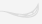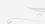Главная

# Mathematical Formulas for Calculation of Newtonian Constant of Gravitation G

How Many Physical Constants are Truly Fundamental? Dr. Nikolay Kosinov Annotation

To main fundamental constants scientists usually refer gravitational constant (G), Planck constant (h) and speed of light (c). These constant are considered to be independent. Researches have shown, that truly fundamental are not constants G, h, c, but quite other constants [1, 2, 3, 4]. They are the following constants:

1. Fundamental quantum of action hu (hu=7,69558071(63) • 10-37 J s).

2. Fundamental length lu (lu=2,817940285(31) • 10-15 m).

3. Fundamental quantum of time tu (tu=0,939963701(11) • 10-23 s).

4. Fine structure constant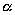(=7,297352533(27) • 10-3 )

5. Number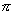(=3,141592653589).

All major fundamental physical constants are composed and consist of these five constant. These five constant have special status and are called "universal superconstants" [1, 2, 3, 4].

Are the major physical constants fundamental?

To main fundamental constant scientists usually refer gravitational constant (G), Planck constant (h) and speed of light (c). These constant are considered to be independent. These physical constants have special status among other constant. It is confirmed by the fact, that in fundamental physics many scientists used the units system, in which these constants equal 1. They received a special importance after M.Planck-s researches, who with the help of their combination discovered the units of mass, length and time, which were called "Planck units".

Constants G, h, c and their different combinations are used in most important physical theories. For example, Newtonian theory of gravitation is G-theory . General theory of relativity is a classic (G, c)-theory. Relative quantum theory of field is quantum (h, c)-by theory . Each of these theories operates by one or two dimensional constants. The discovery of Planck units v Planck length, mass and time - gave a hope, that it is possible to create a new quantum theory on the base of three constants G, h, c. But attempts to create a single quantum theory of electromagnetic fields, particles and gravitation on the base of three dimensional constants G, h, c were unsuccessful. This theory has not existed yet, although scientists showed promises of it . Why did it happen? The reason is, that constants G, h, c can not be a constant base of quantum theory. In accordance with this fact a question arises, whether these constants can be considered primary and independent? Difficulties in creation of G, h, c -theories object it. Obviously, other constant exist, which are both independent, and primary, and truly fundamental. Evidently, all basic physical constants should come of such primary constants, including constants G, h, c. Since primary status of constants G, h, c was out doubts for a long time, the task of search of ontological base of fundamental physical constant does not arise so sharp.

Failures in creation of (G, h, c)-theories and a big number of other fundamental physical constants, among which it is difficult to prefer one of them, bring forward s task of search of ontological base of physical constants. Contemporary physics accumulated already about 300 fundamental constants . 300 constants and all are fundamental! Why such a big number of constants are considered to be fundamental? If we refer them to truly fundamental constants, it exists too much of them. If we accept a fact, that a single material essence makes a world base and all of physical phenomena should have a single nature, then amount of constants must be lesser. Here we remember Ockham-s rule, according to which we should not multiply a number of essences without necessity, and also Fresnel opinion, that L the nature is disposed to operate by great things with the help of the little¦ [5, 8]. Therefore, if priority and independence of constants are criterions of true fundamentality, then just a little amount of constants should be considered to be truly fundamental. That is why, a deep contradiction exists in fact, that hundreds of constants have fundamental status. Our task is to find among them "truly fundamental constants" and reveal a number of them. Many facts indicate, that it is sufficient to have three dimensional constants as truly fundamental. However only three basic units - metre, kilogramme and second v are enough to receive all units, having mechanical nature. But unsuccessful attempts in creation of (G, h, c)-theories show, that three constants are not enough. It means, that we should search the unknown number JF, which comply with a number of still unknown truly fundamental constant, should be searched somewhere between 3 and 300:

3 < JF <300.

Ockham-s principle shows, that we should search right answer of amount of truly fundamental constants nearby 3. The work has been published , and its author comes to the conclusion, that it must be 22 fundamental constants (JF=22). Beneath it will be shown, that their number is much less. We have to find out, whether number JF includes constants G, h, c. We have to find out also, what dimensionless constants can be referred to truly fundamental constants.

Problem of fine structure constant ()

Numerical values of dimensional physical constants depend on selected units system. As it was marked above, by units system selection we can make constants G, h, c become equal 1. Besides that, there are the major dimensionless constants in physics, such as, fine structure constant (= 1/137,03599976(50)), proton-electron mass ratio (mp/me= 1836,1526675(39)) etc. Their values are invariant according to unit-s system selection. The scientists know very little about these constants [11, 13, 14]. Perhaps the only achievement is, that their values are known very exact. Especially enigmatic is fine structure constant ().

Constant () was used in physics by Sommerfeld in 1916, when the theory of fine structure of atom energy was created. Primary fine structure constant () was definite as ratio of electron speed on lower Bohr orbit to speed of light. With the development of quantum theory it become sure, that such simplified conception does not reveal its true sense. The origin nature of this constant and its physical sense has not been discovered yet. Besides fine structure of atom energy, this constant appears in following combination of fundamental physical constants: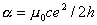. Interesting expression about number (?) belongs to Feinmann : "since it was discovered... it was a riddle. All of tempted physicists-theorists this number brought to deadlock and was followed with anxiety. You wanted to know, how this tie constant appeared: whether it is connected with number π or related to natural logarithm. Noone knows". About the value of fine structure constant the authors of аЕПЙКЕЕБЯЙНЦН physics course write : "we have no theory, which foretold a value of this constant".

In the same time, such invariance of fine structure constant () to unit-s system selection allows to call it the first aspirant after role of truly fundamental constant. Physicists are sure, that fine structure constant () contains something important about structure of microcosm and macrocosm.

Five universal superconstants

As my researches of fundamental physical constants showed [1, 2, 3, 4], that dimensional constants G, h, c are not independent. None of them is primary. It was very unexpected to find out, that gravitational constant (G) is compound [1, 2, 3, 4]. More then that, it was discovered, that gravitational constant (G) includes both Planck constant (h), and speed of light (c) [1, 3, 4]. This is the reason, why constants (G, h, c) can not be a constant base for quantum theory. Therefore it is not strange, that attempts of creation of (G, h, c)-theories are unsuccessful. Interdependent, unprimary, and nonfundamental constants can not be a constant base for fundamental physical theory.

Researches have shown, that truly fundamental are not constants G, h, c, but quite other constants [1, 2, 3, 4]. They are the following constants:

1. Fundamental quantum of action hu (hu=7,69558071(63) • 10-37 J s).

2. Fundamental length lu (lu=2,817940285(31) • 10-15 m).

3. Fundamental quantum of time tu (tu=0,939963701(11) • 10-23 s).

4. Fine structure constant(=7,297352533(27) • 10-3 )

5. Number(=3,141592653589).

They were called universal superconstants, which emphasizes their fundamentality . All physical constants are expressed, using five superconstants hu, lu, tu,,. Table 1 shows some functional dependences for major fundamental physical constants [1, 2, 3, 4]:

## TAB. 1.

 Quantity Symbol Functional dependence Constant of gravitation G G=f(hu,lu,tu,,) Speed of light c c= f(lu,tu) Planck constant H h= f(hu,,) Elementary charge Е e=f(hu,lu,tu) Electron mass me me=f(hu,lu,tu) Rydberg constant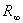=f(lu,,) Proton-electron mass ratio mp/me mp/me=f(,) Hubble constant H0 H0 =f(tu,,) Planck mass mpl mpl=f(hu,lu,tu,,) Planck length lpl lpl=f(lu,,) Planck time tpl tpl=f(tu,,) Magnetic flux quantum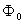=f(hu,lu,tu,,) Bohr magneton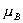= f(hu,lu,tu,)

Researches have shown, that in base of practically all major physical constants these five universal superconstants lie. So, known today family of physical constant assumes reduction to primary superconstant base, because it originates from this primary (hu, lu, tu,,)- basis: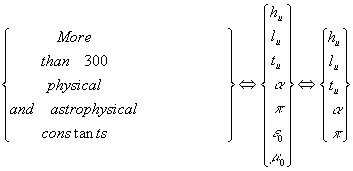As far as magnetic and electric constants do not have physical sense and their introduction is conditioned by only unit-s system selection, physical and astrophysical constants assume reduction to five primary superconstants. Primary, ontological status of universal superconstants allows referring superconstants to separate class of fundamental physical constants. To my mind, we should include to list of fundamental physical constant a new paragraph: "Universal superconstants":

 Universal superconstants Quantity Symbol Value Unit 1 Fundamental quantum hu 7,69558071(63) • 10-37 J s 2 Fundamental length lu 2,817940285(31) • 10-15 m 3 Fundamental time tu 0,939963701(11) • 10-23 s 4 Fine structure constant7,297352533(27) • 10-3 5 Pi3,141592653589...

Emphasizing of special paragraph "Universal superconstants" can be substantiating by following considerations. Five superconstants, included in superconstant basis, are primary constants. All other fundamental physical constants are compound constants and have secondary status. They can be deduced on the base of primary superconstants hu, lu, tu,,. We can deduce by analytic computation practically all major fundamental physical constants, using five superconstants. The author has received proper mathematical correlations for calculation of fundamental physical constant values, using superconstants [1, 2, 3, 4]. To my mind, these five universal superconstants can substitute a big list of electromagnetic, universal, atomic and nuclear constants and become a base of new physical theories of field, elementary particles and gravitation. More detailed information of superconstants you can find on sites:

http://www.sciteclibrary.com/
www.photcoef.com/236.html
www.jsup.or.jp/shiryo/PDF/0900z53.pdf
http://www.rusnauka.narod.ru/
http://www.sciteclibrary.com/rus/catalog/pages/2017.html
http://www.n-t.org/tp/ng/nfk.htm

Ontological status of superconstants hu, lu, tu,,Five superconstants (hu, lu, tu,,) are ontological basis of physical constants. It means, that physical constants originate from five superconstants. All of five superconstants are independent. It is impossible to get dimensionless superconstants, using dimensional superconstants. It is impossible to get dimensional superconstants, using dimensionless superconstants

The principles of superconstant sufficiency for physical constant are formulated in [1, 2]. The first principle of superconstant sufficiency is: "In the base of dimensional fundamental physical constants lie the constants of universal superconstants hu, lu, tu,,group". It is an equivalent formulation: "Values of dimensional fundamental physical constant can be received by calculation, using universal superconstants hu, lu, tu,,".

The second principle of superconstant sufficiency is: "In the base of all dimensionless fundamental physical constants lie two superconstantsand". It is an equivalent formulation: "All dimensionless fundamental physical constants can be received by calculation, using two superconstantsand".

So, five superconstants hu, lu, tu,,are truly fundamental. They have ontological status. The conclusion is, that it was no reason to call other physical constants fundamental

Literature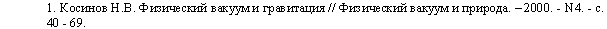2. Kosinov N. Five Fundamental Constants of Vacuum, Lying in the Base of all Physical Laws, Constants and Formulas // Physical Vacuum and Nature. v 2000. - N4. - Я. 96 - 102.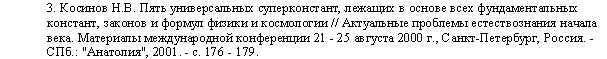4. Kosinov N. V., Kosinova Z. N. GENERAL CORRELATION AMONG FUNDAMENTAL PHYSICAL CONSTANTS //  Journal of New Energy. v 2000. - Vol. 5. v ¦ 1. v Pp. 134 -135.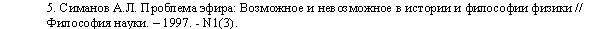6. Peter J., Taylor M. N., Taylor B. N. CODATA Recommended Values of the Fundamental Physical Constants: 1998 //  Reviews of Modern Physihs. v 2000. - Vol 72. - No. 2. - www.physics.nist.gov/constants (Constants in the category "All constants")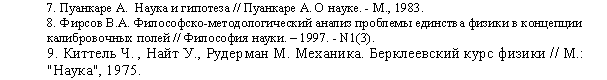10. Carter J. The Other Theory of Physics. - Washington, 1994.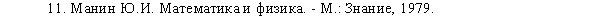12. John Baez. How Many Fundamental Constants Are There? - http://math.ucr.edu/home/baez/constants.html

13. Johnson G. 10 Physics Questions to Ponder for a Millennium or Two // New York Times. v 2000. - Aug. 15

14. Gross D. Millennium Madness: Physics Problems for the Next Millenium. - Strings 2000 conference at University of Michigan. v 2000. - July 10-15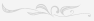## Поиск

Расширенный поиск